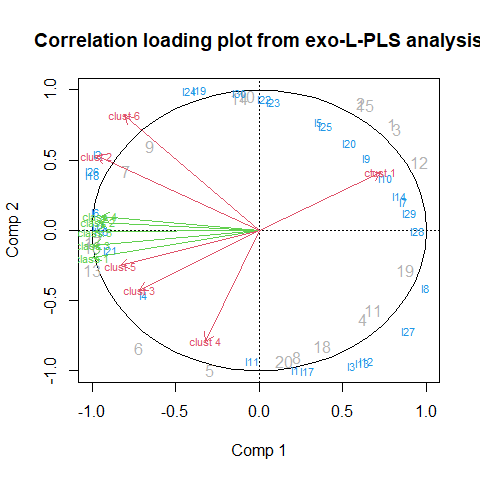F. Complex multiblock analysis

# Start the multiblock R package
library(multiblock)

Complex data structures

The following methods for complex data structures are available in the multiblock package (function names in parentheses):

• L-PLS - Partial Least Squares in L configuration (lpls)
• SO-PLS-PM - Sequential and Orthogonalised PLS Path Modeling (sopls_pm)

L-PLS

To showcase L-PLS we will use simulated data specifically made for L-shaped data. Regression using L-PLS can be either outwards from X1 to X2 and X3 or inwards from X2 and X3 to X1. In the former case, prediction can either be of X2 or X3 given X1. Cross-validation is performed either on the rows of X1 or the columns of X1.

______N
|       |
|       |
|  X3   |
|       |
K|_______|

______N       ________J
|       |     |         |
|       |     |         |
|  X1   |     |   X2    |
|       |     |         |
I|_______|    I|_________|

Simulated L-shaped data

We simulate two latent components in L shape with blocks having dimensions (30x20), (20x5) and (6x20) for blocks X1, X2 and X3, respectively.

set.seed(42)

# Simulate data set
sim <- lplsData(I = 30, N = 20, J = 5, K = 6, ncomp = 2)

# Split into separate blocks
X1  <- sim$X1; X2 <- sim$X2; X3 <- sim$X3 Exo-L-PLS The first L-PLS will be outwards. Predictions have to be accompanied by a direction. # exo-L-PLS: lp.exo <- lpls(X1,X2,X3, ncomp = 2) # type = "exo" is default # Predict X1 pred.exo.X2 <- predict(lp.exo, X1new = X1, exo.direction = "X2") # Predict X3 pred.exo.X2 <- predict(lp.exo, X1new = X1, exo.direction = "X3") # Correlation loading plot plot(lp.exo)Endo-L-PLS The second L-PLS will be inwards. # endo-L-PLS: lp.endo <- lpls(X1,X2,X3, ncomp = 2, type = "endo") # Predict X1 from X2 and X3 (in this case fitted values): pred.endo.X1 <- predict(lp.endo, X2new = X2, X3new = X3) L-PLS cross-validation Cross-validation comes with choices of directions when applying this to L-PLS since we have both sample and variable links. The cross-validation routines compute RMSECV values and perform cross-validated predictions. # LOO cross-validation horizontally lp.cv1 <- lplsCV(lp.exo, segments1 = as.list(1:dim(X1)), trace = FALSE) # LOO cross-validation vertically lp.cv2 <- lplsCV(lp.exo, segments2 = as.list(1:dim(X1)), trace = FALSE) # Three-fold CV, horizontal lp.cv3 <- lplsCV(lp.exo, segments1 = as.list(1:10, 11:20, 21:30), trace = FALSE) # Three-fold CV, horizontal, inwards model lp.cv4 <- lplsCV(lp.endo, segments1 = as.list(1:10, 11:20, 21:30), trace = FALSE) SO-PLS Path Modelling The following example uses the potato data and the wine data to showcase some of the functions available for SO-PLS-PM analyses. Single SO-PLS-PM model A model with four blocks having 5 components per input block is fitted. We set computeAdditional to TRUE to turn on computation of additional explained variance per added block in the model. # Load potato data data(potato) # Single path pot.pm <- sopls_pm(potato[1:3], potato[['Sensory']], c(5,5,5), computeAdditional=TRUE) # Report of explained variances and optimal number of components . # Bootstrapping can be enabled to assess stability. # (LOO cross-validation is default) pot.pm #> direct indirect total additional1 additional2 overall #> 3.23 (2) 49.21 52.44 (3) 4.09 (3) 14.01 (2) 70.55 Multiple paths in an SO-PLS-PM model A model containing five blocks is fitted. Explained variances for all sub-paths are estimated. # Load wine data data(wine) # All path in the forward direction pot.pm.multiple <- sopls_pm_multiple(wine, ncomp = c(4,2,9,8)) # Report of direct, indirect and total explained variance per sub-path. # Bootstrapping can be enabled to assess stability. pot.pm.multiple #>$Smell at rest->View
#>     direct indirect     total
#>  32.68 (1)        0 32.68 (1)
#>
#> $Smell at rest->Smell after shaking #> direct indirect total #> 6.39 (4) 33.64 40.03 (4) #> #>$Smell at rest->Tasting
#>  direct indirect     total
#>   0 (2)    11.52 11.52 (2)
#>
#> $Smell at rest->Global quality #> direct indirect total #> 0 (0) 25.25 25.25 (3) #> #>$View->Smell after shaking
#>     direct indirect     total
#>  30.97 (2)        0 30.97 (2)
#>
#> $View->Tasting #> direct indirect total #> 0.4 (1) 40.69 41.09 (2) #> #>$View->Global quality
#>  direct indirect     total
#>   0 (0)    30.87 30.87 (2)
#>
#> $Smell after shaking->Tasting #> direct indirect total #> 56.67 (3) 0 56.67 (3) #> #>$Smell after shaking->Global quality
#>  direct indirect     total
#>   0 (2)    70.15 70.15 (2)
#>
#> \$Tasting->Global quality
#>     direct indirect     total
#>  78.12 (2)        0 78.12 (2)